# KR101357690B1 - The calculation method of interferometer array antenna spacing ratios for direction finder - Google Patents

The calculation method of interferometer array antenna spacing ratios for direction finder Download PDF

## Info

Publication number
KR101357690B1
KR101357690B1 KR1020120114951A KR20120114951A KR101357690B1 KR 101357690 B1 KR101357690 B1 KR 101357690B1 KR 1020120114951 A KR1020120114951 A KR 1020120114951A KR 20120114951 A KR20120114951 A KR 20120114951A KR 101357690 B1 KR101357690 B1 KR 101357690B1
Authority
KR
South Korea
Prior art keywords
phase difference
array
method
antenna
calculating
Prior art date
Application number
KR1020120114951A
Other languages
Korean (ko)
Inventor
이정훈
주증민
Original Assignee
국방과학연구소
Priority date (The priority date is an assumption and is not a legal conclusion. Google has not performed a legal analysis and makes no representation as to the accuracy of the date listed.)
Filing date
Publication date
Application filed by 국방과학연구소 filed Critical 국방과학연구소
Priority to KR1020120114951A priority Critical patent/KR101357690B1/en
Application granted granted Critical
Publication of KR101357690B1 publication Critical patent/KR101357690B1/en

• 238000004364 calculation methods Methods 0.000 title claims description 5
• 230000001131 transforming Effects 0.000 claims description 34
• 238000000034 methods Methods 0.000 description 1

## Images

•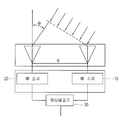••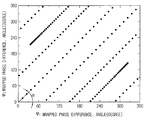•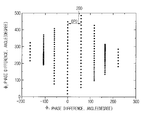•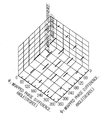•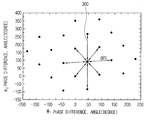•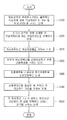## Classifications

• GPHYSICS
• G01MEASURING; TESTING
• G01S3/00Direction-finders for determining the direction from which infrasonic, sonic, ultrasonic, or electromagnetic waves, or particle emission, not having a directional significance, are being received
• G01S3/02Direction-finders for determining the direction from which infrasonic, sonic, ultrasonic, or electromagnetic waves, or particle emission, not having a directional significance, are being received using radio waves
• G01S3/14Systems for determining direction or deviation from predetermined direction
• G01S3/46Systems for determining direction or deviation from predetermined direction using antennas spaced apart and measuring phase or time difference between signals therefrom, i.e. path-difference systems
• GPHYSICS
• G01MEASURING; TESTING
• G01S3/00Direction-finders for determining the direction from which infrasonic, sonic, ultrasonic, or electromagnetic waves, or particle emission, not having a directional significance, are being received
• G01S3/02Direction-finders for determining the direction from which infrasonic, sonic, ultrasonic, or electromagnetic waves, or particle emission, not having a directional significance, are being received using radio waves
• G01S3/14Systems for determining direction or deviation from predetermined direction
• G01S3/46Systems for determining direction or deviation from predetermined direction using antennas spaced apart and measuring phase or time difference between signals therefrom, i.e. path-difference systems
• G01S3/48Systems for determining direction or deviation from predetermined direction using antennas spaced apart and measuring phase or time difference between signals therefrom, i.e. path-difference systems the waves arriving at the antennas being continuous or intermittent and the phase difference of signals derived therefrom being measured

## Abstract

A method for calculating spacing ratio of an interferometer arrangement antenna for direction detection comprises a step for setting the distance between an azimuth angle and a phase difference of instantaneous field of view (IFOV) for signals received to an arrangement antenna and calculating the number of phase difference lines and phase difference vectors for the phase difference of the signals outputted from a phase comparator of a direction detection device; a step for performing a modular operation for the phase difference vectors and calculating a phase difference matrix; a step for calculating a conversion matrix and performing linear conversion with the conversion matrix; a step for calculating the distance between the phase differences on a new axis; and a step for calculating an antenna interval with an arrangement interval of the arrangement antenna. [Reference numerals] (10,20) RF device; (30) Phase comparator

## Description

The calculation method of interferometer array antenna spacing ratios for direction finder}

One embodiment of the present invention relates to a method for calculating a separation ratio of an interferometer array antenna for performing phase comparison direction detection.

A general direction finder is a receiving device that measures a direction in which radio waves arrive, and is also called a wireless direction finder. Unlike radar, direction finders do not emit radio waves. Directional detectors are used for the safety of ships and aircrafts, and are being used for clarification or monitoring of illegal propagation.

Such a direction detector is composed of an antenna for receiving radio waves and a receiver for processing the received radio waves. The structure of the antenna and receiver of the direction finder has various structures and shapes by the direction detecting method.

Various direction detection technologies include rotation direction detection technology, amplitude comparison direction detection technology, and phase comparison direction detection technology called interferometer technology.

Interferomer technology uses phase comparison to detect direction. This is a technique used to highly accurately position the emitter in the wide frequency range from direct current to light. The components of the interferometer are two matching antennas and a receiver. The radio waves received from the interferometer by the two-channel antenna are converted to intermediate frequencies at each receiver and transmitted to the phase comparator. Here, relative phase angles along the direction of arrival of the two signals are measured. The relative phase angle is transmitted to the anti-virus processor, i.e., the processor, where the angle of arrival (AOA) of the radio wave relative to the baseline connecting the two antenna directions is calculated. In a typical interferometer system, the processor accepts baseline orientation information (true north or horizontal plane) to determine true heading or elevation for the emitter.

The interferometer array antenna according to the present invention determines an azimuth angle of a received signal by using a state phase difference measured between antennas with an antenna system composed of two or more numbers. In particular, the azimuth angle of the radio signal source is estimated by comparing the phase of the radio signal source to estimate the angle of arrival of the radio signal source including the equipment of electronic warfare such as early warning threat detection, tracking and electronic information. When spacing an interferometer array to obtain high accuracy, the spacing of the antennas to avoid ambiguity and to meet the required accuracy of direction detection is one of the important factors.

The antenna spacing is obtained by probability calculation that minimizes ambiguity in consideration of the electrical phase error component due to the signal-to-noise ratio and the error component due to the electrical phase mismatch of the equipment, or the phase difference set output by the array antenna does not overlap in IFOV. It can be found using mutually exclusive means.

The first method is complicated and the second method is simple, but the phase difference occurring in the room detector is not considered.

Therefore, there is a need for a method of calculating the array spacing of the direction detectors by supplementing these methods.

An object of the present invention is to provide a method for obtaining a separation ratio of an interferometer array antenna for performing phase comparison direction detection.

In order to achieve the above object of the present invention, the method of calculating the inter-antenna array interval of the interferometer array antenna according to an embodiment of the present invention, the instantaneous maximum viewing angle (IFOV) of the signals received by the array antenna Setting the azimuth and the phase difference distance, obtaining the number of phase difference lines and phase difference vectors for the phase difference of the signals output from the phase comparator of the direction detecting device, and performing a modular operation on the phase difference vectors. Obtaining a phase difference matrix, obtaining a transformation matrix for linearly transforming the phase difference matrix, linearly transforming the transformation matrix, obtaining a distance between each phase difference in a new axis generated by the linear transformation, and each phase difference The antenna spacing between the arrays of array antennas whose distance between Calculating the price.

According to an example related to the present invention, when the array antenna is a 3-element linear array, two phase differences output from the phase comparator may be used, and when the 4-element linear array is used, three phase differences may be used.

According to an example related to the present invention, the phase difference vector is

Using The phase difference vector is It can be made in multiples of.

According to an example related to the present invention, the azimuth angle of the instantaneous maximum viewing angle (IFOV)

Can be set within

According to an example related to the present invention, the modular operation is the instantaneous maximum viewing angle in the three-element array and four-element array

In the realm And the instantaneous maximum viewing angle In the realm Can be performed.

According to an embodiment related to the present invention, the linear transformation is a mathematical expression in a three-element array

Can be used.

According to an example related to the present invention, the transformation matrix is

Where α is Can be satisfied.

According to an example related to the present invention, the linear transformation is performed in a four-element array.

Can be used.

According to an example related to the present invention, the transformation matrix is

, Where α and β are , Can be satisfied.

According to an example related to the present invention, the distance between phase differences is

, , Can be used.

According to an example related to the present invention, in order to satisfy a value in which the distance between each phase difference is greater than a desired distance between phase differences R,

Can be used.

The method for calculating the interferometer array antenna separation ratio for direction detection according to the present invention can more easily set the antenna spacing of the array antenna despite the influence of the complexity of the system or the mounting platform.

1 is a block diagram of a two-element interferometer direction detection device.
FIG. 2A is a phase difference diagram of two phase difference outputs in a three-element linear array (when no modular conversion is performed). FIG.
2B is a phase difference diagram when two phase difference outputs are performed in a three-element linear array (when modular conversion is performed).
Figure 2c is a phase difference diagram of the linear conversion of the two phase difference output in the three-element linear array.
3A is a phase difference diagram of three phase difference outputs in a four-element linear array (when modular conversion is performed).
Figure 3b is a phase difference diagram of the linear conversion for the three phase difference output in the four-element linear array.
Figure 4 is a flow chart of a method for calculating the interferometer array antenna separation ratio for direction detection according to the present invention.

Hereinafter, a method for calculating an interferometer array antenna separation ratio for direction detection according to the present invention will be described in more detail with reference to the accompanying drawings. The suffix "module" and " part "for the components used in the following description are given or mixed in consideration of ease of specification, and do not have their own meaning or role. In the present specification, the same or similar reference numerals are given to different embodiments in the same or similar configurations. As used herein, the singular forms "a", "an" and "the" include plural referents unless the context clearly dictates otherwise.

The present invention is applied to a method for obtaining an interferometer array antenna spacing for performing phase comparison direction detection.

The phase comparison direction detection apparatus estimates the direction by comparing the phase difference of the received radio signal. 1 illustrates an interferometer array antenna using two general antennas. The signal received by the antenna passes through RF components such as the RF elements 10 and 20 and then outputs the phase difference between the two antennas in the phase comparator 30. The phase difference output at this time is given by Equation 1.

Where λ is the wavelength, d is the distance between the antennas, and θ is the incident angle of the unsigned signal. In addition, since the phase difference is repeated every ± 2π, the phase difference of the multi-interferometer having a plurality of antennas can be represented by Equation 2.

Where fix (x) is the integer closest to zero, rounded up to x. And instantaneous field of view (IFOV)

. In addition, since the electrical phase angle φ is output by modulo (mod2π) in the phase comparator, it is given by Equation (3).

Where n is the antenna spacing index. ψ is a phase difference output after modulo (mod2π) by the phase comparator 30. In the interferometer linear array using n + 1 antennas, each phase difference ratio is represented by Equation 4 by Equation 3.

When the modular transformation is not performed, the phase difference diagram for the two phase difference outputs in the three element linear array (an array antenna formed with three antenna elements) is shown in FIG. 2A.

Hereinafter, a method of calculating a separation ratio between antenna elements of an interferometer array antenna for direction detection according to an embodiment of the present invention will be described.

The first step (S100) for calculating the separation ratio of the interferometer array antenna is to obtain a modular constant and a phase difference vector of each phase difference output from the phase comparator 30 of the direction detecting apparatus. The modular constant of each phase difference can be calculated using Equation 2.

2b is a phase difference

And About A phase difference plan view performing a modular operation. In Fig. 2a, the phase difference or Since is output after the modular operation, a phase difference line is generated whenever the phase difference is a multiple of ± 2π. Therefore, phase difference Each time is a multiple of ± 2π Can be obtained using Equation 4. Also, phase difference Whenever becomes ± 2π, the corresponding phase difference Can be obtained using Equation 4.

In order to calculate the unambiguous array spacing, the lines generated after the modular operation should not overlap, and the distance of the lines should satisfy the phase difference required by the DF. To do this, after reconstructing the phase difference for each phase difference line to a new coordinate system, the array spacing is obtained from the new coordinate system. In addition, the number of lines generated by the phase difference can be obtained by Equation 2. Phase difference in equation (2)

Integer for Phase difference Integer for to be.

To obtain the phase difference data, IFOV is divided into two regions based on 0 [degrees], and 0 is

Wow .

IFOV

Phase difference from equation (2) in domain Wow An integer corresponding to Wow Can be obtained. When IFOV is maximum, each output phase difference , Therefore, the number of phase difference lines generated in this region to be. Also, When the number of the same line is the same.

Therefore, IFOV

The total number of phase difference lines produced in the region And the required number of phase difference data is the same.

2 in 3 element linear array Phase difference  If you use output

IFOV

Phase difference in the area silver Repeat 2π times. therefore, The phase difference vector including And the corresponding phase difference is .

Phase difference

silver To Repeat times. Therefore, the phase difference vector And the corresponding phase difference is .

IFOV

Phase difference in the area silver time . therefore, The phase difference vector including And the corresponding phase difference is .

Phase difference

silver To Repeat times. Therefore, the phase difference vector And the corresponding phase difference is .

3 in 4 element linear array Phase difference  If you use output

IFOV

In the area

Phase difference

silver To Repeat times. therefore, The phase difference vector including And the corresponding phase difference is And .

Phase difference

silver To Repeat times. Therefore, the phase difference vector And the corresponding phase difference is And .

Phase difference

The To Repeat times. Therefore, the phase difference vector And the corresponding phase difference is And .

IFOV

In the area

Phase difference

silver To Repeat times. therefore, The phase difference vector including And the corresponding phase difference is And .

Phase difference

silver To Repeat times. Therefore, the phase difference vector And the corresponding phase difference is And .

Phase difference

The To Repeat times. Therefore, the phase difference vector And the corresponding phase difference is And .

The second step S200 is to perform a modular operation on each phase difference vector generated by the antenna spacing.

As shown in Fig. 1, the illustrated phase comparator 30

Modulo operation is performed to output the phase difference. That is, the phase comparator outputs the unwrapped phase difference of FIG. 2A as the wrapped phase difference as shown in FIG. 2B. Accordingly, the phase difference vector may be obtained by performing a modular transformation on the phase difference vector.

For phase difference vectors using a three-element array, IFOV

Phase difference vector obtained from the domain For each By doing, Wrapped Find the phase difference vector of.

IFOV

The phase difference found in the domain is IFOV Phase difference vector because it is generated inverse to the phase difference in For each By doing, Wrapped Find the phase difference vector.

In addition, IFOV is obtained for a phase difference vector using three phase differences using a four-element array.

Phase difference vector obtained from the domain , , For each By doing, Wrapped , , Find the phase difference vector of.

IFOV

The phase difference found in the domain is IFOV Phase difference vector , , For each By doing, Wrapped , , Find the phase difference vector of.

The third step (S210) is a step of obtaining a phase difference matrix from a phase difference vector.

In the case of a three-element linear array, the wrapped phase difference vector may be represented as a matrix by the phase difference vectors of the X and Y axes for linear transformation.

In the case of a four-element linear array, a phase difference vector of a Z-axis may be added for linear transformation and represented as a phase difference matrix.

The fourth step (S300) is a step of obtaining a transformation matrix to linearly transform each phase difference matrix.

FIG. 2B shows the wrapped phase difference. In this case, the inclination of the straight line generated by the phase difference is α, and if the rotation is made counterclockwise by this inclination, it can be obtained as shown in FIG. 2C. Since the phase difference matrix can be rotated by the inclination angle α, The transformation matrix is shown in equation (7).

here,

to be.

In addition, in the four antenna array, the phase difference line shown in FIG. 3A uses an Euler angle to enable the conversion to the phase difference point as shown in FIG. 3B. Phase difference

In this case, the transformation matrix applied to the four element arrays is represented by Equation (8).

here

, to be.

The fifth step (S400) is a step of linearly transforming the phase difference matrix using the transform matrix.

In the three antenna arrays, the phase difference matrix of Equation 5 is linearly transformed by the transformation matrix of Equation 7, which is represented by Equation 9 below.

If the axial transformation is performed on the phase difference matrix sampled from the three antenna arrays, a phase difference straight line can be obtained as shown in FIG. 2C, and the elements of the matrix V consist of phase differences generated in a new coordinate system. In FIG. 2C, since the horizontal axis represents ambiguity and the vertical axis represents the phase difference resolution of the direction detector, the second row vector in the matrix V of Equation 8 is not related to the ambiguity. Therefore, if a value is selected such that the distance of the first row vector element of the matrix V is greater than or equal to a certain value, the interferometer direction detecting device using the three-element array does not cause ambiguity.

In the four antenna arrays, the phase difference matrix of Equation 6 is linearly transformed by the transform matrix of Equation 8 and is represented by Equation 10.

The axial transformation of the phase difference matrix sampled at the four antenna arrays generated several phase difference lines as shown in Fig. 3a. A linearized matrix

Figure 3b is shown in Figure 3b, Figure 3b after the linear transformation ( A phase difference diagram projected on a plane, and a straight line is projected as a point. Thus, as with the three antenna arrays ( The point and the point distance generated by the phase difference on the plane represent the ambiguity interval, Since the phase difference corresponding to the axis represents the phase difference resolution of the direction detecting apparatus, it can be seen that the third row vector in the matrix V of Equation 9 is not related to ambiguity. Therefore, if the value represented by the first and second points of the matrix V and the distance between the points is selected to be greater than or equal to a certain value, the interferometer direction detecting device using the four-element array does not cause ambiguity.

The sixth step S500 is to calculate a distance between phase difference (DPD) in a new axis generated by the linear transformation.

As shown in Figs. 2C and 3B, the three-element array has a distance between phase retardation 200 of each phase difference line in a new axis by linear transformation, and the four-element array has each phase difference point in a new axis by linear transformation. Distance 300 is the phase difference distance DPD. The interval between each phase difference is shown in Equation (11).

,

Here, if l is 1, it is a 3 element array, and if l is 2, it is a 4 element array.

The seventh step S600 is to obtain an antenna interval where each interphase difference satisfies a value greater than a desired interphase difference R. This is represented by Equation 12. That is, the distance between phases satisfying the following equation is calculated as the antenna spacing between elements of the array antenna.

This completes the method of calculating the interferometer array antenna separation ratio.

Table 1 summarizes the arrangement intervals for the interferometer array antennas using 3 and 4 elements by using the direction detecting interferometer array antenna separation ratio calculation method according to the present invention.

Three element array

Four element array
0.5
0.6
0.7
0.7
0.8
0.8
0.8
0.9
0.9
0.9
0.9
0.9
0.9
1.0
2.9
2.1
2.4
2.5
2.0
2.1
2.8
2.1
2.2
2.3
2.4
3.1
3.2
2.3
48.9
49.4
43.2
41.6
66.8
48.0
49.4
47.2
60.5
58.3
42.1
44.6
43.3
43.0
0.7
0.7
0.7
0.8
0.8
0.8
0.8
0.8
0.9
0.9
0.9
0.9
0.9
0.9
1.0
1.0
1.0
1.5
2.0
2.0
2.5
3.0
1.5
1.5
2.0
2.0
2.5
3.0
2.5
3.5
4.5
2.0
2.5
4.5
3.0
3.5
2.0
3.5
2.5
3.5
3.0
3.5
91.5
88.5
89.4
88.9
103.5
85.7
92.5
87.4
123.4
96.5
112.5
89.3
106.3
104.8

The maximum value of the viewing angle

end In the case of the interferometer direction detector using a three-element array, the distance between the phase difference (R) is 40 [degree] and when the separation ratio is calculated according to the present invention, the distance of the antenna satisfying this is shown on the left side of Table 1. As shown.

This means that in the case of a three-element array antenna, the separation ratio between antenna elements is as follows. That is, the distance d 1 between the first antenna element and the second antenna element is 0.5 times the wavelength corresponding to the center frequency radiated through the antenna, and the distance d between the second antenna element and the third antenna element If 2 ) is 2.9 times the wavelength corresponding to the center frequency radiated through the antenna, the phase difference is 48.9 [degree], so the separation ratio between antenna elements is satisfied.

In addition, in the case of the interferometer direction detector using a four-element array, the distance between phase differences (R) is 80 [degree] and the distance between antenna elements satisfying this when the separation ratio is calculated according to the present invention is shown on the right side of Table 1. As shown.

Here, d 1 , d 2, and d 3 correspond to the distance between the first antenna element and the second antenna element, the distance between the second antenna element and the third antenna element, and the distance between the third antenna element and the first antenna element, respectively.

The above-described method for calculating the interferometer array antenna spacing ratio for direction detection is not limited to the configuration and method of the above-described embodiments, and the above embodiments may be modified in various ways so that various modifications may be made. All or part may be optionally combined.

<Explanation of symbols for the main parts of the drawings>

10, 20: RF element 30: phase comparator

## Claims (11)

1. In the method of calculating the inter-antenna array spacing of the interferometer array antenna,
Setting azimuth and phase difference distances of the instantaneous maximum viewing angle (IFOV) with respect to signals received by the array antenna, and obtaining phase difference vectors with respect to the phase difference of signals output from the phase comparator of the direction detecting device;
Performing a modular operation on the phase difference vectors and using the same to obtain a phase difference matrix;
Obtaining a transformation matrix for linearly transforming the phase difference matrix and performing linear transformation using the transformation matrix;
Obtaining a distance between each phase difference in a new axis generated by the linear transformation; And
A method for calculating the antenna spacing between interferometer array antennas, comprising: calculating an antenna spacing for each phase difference distance greater than a desired phase difference distance as an array spacing of array antennas.
2. The method of claim 1,
When the array antenna is a three-element linear array, two phase differences output from the phase comparator are used, and when the four-element linear array is used, three phase differences are used. Interval calculation method.
3. The method of claim 3,
Azimuth angle of the instantaneous maximum viewing angle (IFOV) A method of calculating an array interval between antennas of an interferometer array antenna, characterized in that set within.
4. The method of claim 1,
The linear transformation is an equation in a three-element array Method for calculating the array interval between antennas of the interferometer array antenna, characterized in that using.
5. The method according to claim 6,
The transformation matrix is Where α is Method for calculating the array interval between antennas of the interferometer array antenna, characterized in that satisfying the.
6. The method of claim 1,
The linear transformation is a four element array Method for calculating the array interval between antennas of the interferometer array antenna, characterized in that using.
7. The method of claim 1,
In order to satisfy the value that the distance between phases is larger than the desired distance between phases, Method for calculating the array interval between antennas of the interferometer array antenna, characterized in that using.
KR1020120114951A 2012-10-16 2012-10-16 The calculation method of interferometer array antenna spacing ratios for direction finder KR101357690B1 (en)

## Priority Applications (1)

Application Number Priority Date Filing Date Title
KR1020120114951A KR101357690B1 (en) 2012-10-16 2012-10-16 The calculation method of interferometer array antenna spacing ratios for direction finder

## Applications Claiming Priority (2)

Application Number Priority Date Filing Date Title
KR1020120114951A KR101357690B1 (en) 2012-10-16 2012-10-16 The calculation method of interferometer array antenna spacing ratios for direction finder
US13/936,288 US9310458B2 (en) 2012-10-16 2013-07-08 Method for calculating spacing ratio of interferometer array antenna for direction finder

## Publications (1)

Publication Number Publication Date
KR101357690B1 true KR101357690B1 (en) 2014-02-04

# Family

## Family Applications (1)

Application Number Title Priority Date Filing Date
KR1020120114951A KR101357690B1 (en) 2012-10-16 2012-10-16 The calculation method of interferometer array antenna spacing ratios for direction finder

## Country Status (2)

US (1) US9310458B2 (en)
KR (1) KR101357690B1 (en)

## Cited By (6)

* Cited by examiner, † Cited by third party
Publication number Priority date Publication date Assignee Title
KR101616377B1 (en) 2014-04-24 2016-05-11 국방과학연구소 Electronic support measures phase interferometer direction finding system for the direction accuracy improvement
KR101638481B1 (en) 2015-01-09 2016-07-11 국방과학연구소 Method and Apparatus for correcting interferometer of array antenna with tilt angle
KR20180083174A (en) * 2017-01-12 2018-07-20 국방과학연구소 Apparatus and method for detecting direction of arrival signal in Warfare Support System
KR20180113267A (en) * 2017-04-06 2018-10-16 서울대학교산학협력단 Method and apparatus for estimating direction of arrival using generation of virtual received signals based on uniform linear array antenna
KR101952370B1 (en) 2018-04-16 2019-02-26 국방과학연구소 Method for determining an antena array spacing, apparatus therefor and computer readable storage medium
KR20190043023A (en) * 2017-10-17 2019-04-25 국방과학연구소 The method for calculating ambiguity probability of the direction of arrival

## Families Citing this family (3)

* Cited by examiner, † Cited by third party
Publication number Priority date Publication date Assignee Title
CN104330767A (en) * 2014-11-27 2015-02-04 中国船舶重工集团公司第七二四研究所 Interferometer direction-finding method based on phase fuzzy number search and least square fit
KR101603142B1 (en) * 2015-04-23 2016-03-14 국방과학연구소 Method for obtaining the multiple element array spacing for phase interferometer direction finder
RU2634299C1 (en) * 2016-12-08 2017-10-26 Федеральное государственное бюджетное образовательное учреждение высшего образования "Московский технологический университет" Phase radio direction-finding apparatus

## Citations (4)

* Cited by examiner, † Cited by third party
Publication number Priority date Publication date Assignee Title
KR101032299B1 (en) 2011-01-03 2011-05-06 삼성탈레스 주식회사 Self-calibration for direction finding in multi-baseline interferometer system
KR20110049113A (en) * 2009-11-04 2011-05-12 인하대학교 산학협력단 Average internal loop-back antenna calibration method for array antenna system
KR20110094857A (en) * 2010-02-18 2011-08-24 삼성전자주식회사 Method and device for estimating angle of arrival
KR20110113926A (en) * 2010-04-12 2011-10-19 한국과학기술원 Synthetic aperture radar system for continuous wide swath high resolution imaging and method thereof

## Family Cites Families (3)

* Cited by examiner, † Cited by third party
Publication number Priority date Publication date Assignee Title
US6489923B1 (en) * 1999-09-16 2002-12-03 Nortel Networks Limited Position location method and apparatus for a mobile telecommunications system
US20060082501A1 (en) * 2004-10-15 2006-04-20 Interdigital Technology Corporation Method and apparatus for direction finding using phase comparison
US7213442B2 (en) * 2004-10-18 2007-05-08 The Boeing Company Methods of arranging transducers and transducer arrays having reduced phase ambiguity for determining direction of arrival of received signals

## Patent Citations (4)

* Cited by examiner, † Cited by third party
Publication number Priority date Publication date Assignee Title
KR20110049113A (en) * 2009-11-04 2011-05-12 인하대학교 산학협력단 Average internal loop-back antenna calibration method for array antenna system
KR20110094857A (en) * 2010-02-18 2011-08-24 삼성전자주식회사 Method and device for estimating angle of arrival
KR20110113926A (en) * 2010-04-12 2011-10-19 한국과학기술원 Synthetic aperture radar system for continuous wide swath high resolution imaging and method thereof
KR101032299B1 (en) 2011-01-03 2011-05-06 삼성탈레스 주식회사 Self-calibration for direction finding in multi-baseline interferometer system

## Cited By (9)

* Cited by examiner, † Cited by third party
Publication number Priority date Publication date Assignee Title
KR101616377B1 (en) 2014-04-24 2016-05-11 국방과학연구소 Electronic support measures phase interferometer direction finding system for the direction accuracy improvement
KR101638481B1 (en) 2015-01-09 2016-07-11 국방과학연구소 Method and Apparatus for correcting interferometer of array antenna with tilt angle
KR20180083174A (en) * 2017-01-12 2018-07-20 국방과학연구소 Apparatus and method for detecting direction of arrival signal in Warfare Support System
KR101957291B1 (en) * 2017-01-12 2019-03-12 국방과학연구소 Apparatus and method for detecting direction of arrival signal in Warfare Support System
KR20180113267A (en) * 2017-04-06 2018-10-16 서울대학교산학협력단 Method and apparatus for estimating direction of arrival using generation of virtual received signals based on uniform linear array antenna
KR101953697B1 (en) * 2017-04-06 2019-03-04 서울대학교산학협력단 Method and apparatus for estimating direction of arrival using generation of virtual received signals based on uniform linear array antenna
KR20190043023A (en) * 2017-10-17 2019-04-25 국방과학연구소 The method for calculating ambiguity probability of the direction of arrival
KR102022589B1 (en) * 2017-10-17 2019-09-18 국방과학연구소 The method for calculating ambiguity probability of the direction of arrival
KR101952370B1 (en) 2018-04-16 2019-02-26 국방과학연구소 Method for determining an antena array spacing, apparatus therefor and computer readable storage medium

## Also Published As

Publication number Publication date
US9310458B2 (en) 2016-04-12
US20140104111A1 (en) 2014-04-17

## Similar Documents

Publication Publication Date Title
US10571544B2 (en) Direction finding using signal power
DE102004033375B4 (en) Circular super-directing receive antenna arrays
US7295150B2 (en) Methods and systems for identifying high-quality phase angle measurements in an interferometric radar system
US5561432A (en) Out of plane antenna vector system and method
Wen et al. Angle estimation and mutual coupling self-calibration for ULA-based bistatic MIMO radar
US20050062647A1 (en) Direction finding method and system using digital directional correlators
US5574468A (en) Phase-equivalent interferometer arrays
US6061022A (en) Azimuth and elevation direction finding system based on hybrid amplitude/phase comparison
US4996533A (en) Single station radar ocean surface current mapper
CN107024621B (en) Massive-MIMO antenna measurement device and its directivity measurement method
US7136012B2 (en) Approach radar with array antenna having rows and columns skewed relative to the horizontal
CN1037399A (en) Method and apparatus in radio theodolite antenna and the receiving system
WO2008099390A2 (en) Indoor location determination
US4209791A (en) Antenna apparatus for bearing angle determination
US6255991B1 (en) Low cost angle of arrival measurement system
JP2015505043A (en) Method and sensor for determining the direction of arrival of incident radiation
US7466262B2 (en) Positioning system with a sparse antenna array
US7026990B2 (en) Interferometer arrangement for unambiguous determination of an angle of incidence of incident electromagnetic radiation
US9279884B2 (en) Method and device for estimating direction of arrival
US9024812B2 (en) Systems and methods for providing antenna calibration
US20160116582A1 (en) Radar imaging via spatial spectrum measurement and MIMO waveforms
US6169519B1 (en) TCAS bearing measurement receiver apparatus with phase error compensation method
KR20190130990A (en) Compact 3d direction finder

## Legal Events

Date Code Title Description
A201 Request for examination
E902 Notification of reason for refusal
E701 Decision to grant or registration of patent right
GRNT Written decision to grant
FPAY Annual fee payment

Payment date: 20170102

Year of fee payment: 4

FPAY Annual fee payment

Payment date: 20190102

Year of fee payment: 6

FPAY Annual fee payment

Payment date: 20200103

Year of fee payment: 7